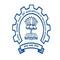Precision Handling in Python

# Precision Handling in Python

clickHere
Updated on Oct 31, 2022 18:28 IST

Python comes with a set of functions that can be used for the precision handling of decimal (floating point) numbers. Most of these functions are under the umbrella of the math module in python. In this article, we will look into various functions and operators used for precision handling in python as listed below:

Let’s take a look at each one of them in detail.

Compare best Python Courses

## Using trunc() Function:

The trunc() function in python is used to eliminate the fractional or decimal part of a floating-point number. The trunc() function belongs to python’s math module, therefore to use it we need to first import the math module into our program.

Syntax:

`math.trunc(float_value)`

Arguments:

• float_value: It takes a floating point number as an argument.

Returns: The trunc() function returns the integer of a floating point number.

Example: Using trunc() function for precision handling.

` `
```# import the math moduleimport math # declare a float variablefloat_value = 101.101 #use the trunc() functionprecision_value = math.trunc(float_value) # print the precision valueprint(precision_value)Copy code```

Output:

`101`

As you can observe in the above output, the fractional part of the floating value is removed once the trunc() function is used upon it.

## Using ceil() Function:

The celi() function in python is used to return the smallest integer greater than the given floating-point number. The celi()  function belongs to python’s math module, therefore to use it we need to first import the math module into our program.

Syntax:

`math.ceil(float_value)`

Arguments:

• float_value: It takes a floating point number as an argument.

Returns: The ceil() function returns the smallest integer greater than the floating point number.

Example: Using ceil() function for precision handling.

` `
```# import the math moduleimport math # declare a float variablefloat_value = 101.101 #use the celi() functionprecision_value = math.ceil(float_value) # print the precision valueprint(precision_value)Copy code```

Output:

`102`

As you can observe in the above output, the least integer greater than the float value is returned as the ceil() function is used upon it.

## Using floor() Function:

The floor() function in python is used to return the largest integer smaller than the given floating-point number. The floor()  function belongs to python’s math module, therefore to use it we need to first import the math module into our program.

Syntax:

`math.floor(float_value)`

Arguments:

• float_value: It takes a floating point number as an argument.

Returns: The floor() function returns the largest integer smaller than the floating point number.

Example: Using floor() function for precision handling.

` `
```# import the math moduleimport math # declare a float variablefloat_value = 101.101 # use the celi() functionprecision_value = math.floor(float_value) # print the precision valueprint(precision_value)Copy code```

Output:

`101`

As you can observe in the above output, the largest integer smaller than the float value is returned as the floor() function is used upon it.

## Using format() Function:

The format() function in python can be used to format a float value for precision based on the format specifier.

Syntax:

`format_specifier.format(value)`

Arguments:

• value: In the above syntax, the value represents the entity that is to be formatted.
• format_specifier: The format specifier in the above syntax specifies how the value is to be formatted.

Returns: The format() function returns a formatted string based on the format specifier.

Example: Using format() function for precision handling.

` `
```# declare a float variablefloat_value = 101.101505 # use the format() function to# format the foat value upto# three decimal placesprecision_value = '{0:.3f}'.format(float_value)) # print the precision valueprint(precision_value)Copy code```

Output:

`101.102`

As you can observe in the above output, the value up to 3 decimal places are returned as the format() function is used upon it.

Note: The format() function can also be used to format a string as per the format specifier.

## Using round() Function:

The round() function in python can be used to format a float value or round off a float point value up to n decimal places.

Syntax:

`round(value,n)`

Arguments:

• value: In the above syntax, the value represents the entity that is to be formatted.
• n: The n in the above syntax specifies the decimal palces upto which the rounding off is to be done.

Returns: The round() function returns rounded-off float values based on the given value of n.

Example: Using round() function for precision handling.

` `
```# declare a float variablefloat_value = 101.101505 # use the round() function to# format the foat value upto# three decimal placesprecision_value = round(float_value,3) # print the precision valueprint(precision_value)Copy code```

Output:

`101.102`

As you can observe in the above output, the value up to 3 decimal places are returned as the round() function is used upon it.

## Using % Operator:

The % operator in python can also be used to set precision over a float value based upon the precision specifier similar to that of the format() function.

Syntax:

`'%.nf' %value`

Arguments:

• value: In the above syntax, the value represents the entity that is to be formatted.
• n: The n in the above syntax specifies the decimal places up to which the rounding off is to be done.

Returns: The % operator returns rounded-off float values based on the given value of n.

Example: Using % operator for precision handling.

` `
```# declare a float variablefloat_value = 101.101505 # use the % operator to# format the foat value upto# three decimal placesprecision_value = '%.3f' %float_value # print the precision valueprint(precision_value)Copy code```

Output:

`101.102`

As you can observe in the above output, the value up to 3 decimal places are returned as the % operator is used upon it.

## Conclusion

In this article, we have managed to cover 5 different ways that we can handle precision in python. The aim of this article is to provide you with all possible solutions that can be used for precision handling in python based on your needs. Please comment if you find any mistakes or irregularities in the article. Give it a thumbs up if you enjoyed the read.

clickHere

This is a collection of insightful articles from domain experts in the fields of Cloud Computing, DevOps, AWS, Data Science, Machine Learning, AI, and Natural Language Processing. The range of topics caters to upski... Read Full Bio

## Trending Technology CoursesPython Training
IIT Bombay4.2Python Fundamentals for Beginners
Great Learning4.5Programming for Everybody (Getting Started with Python)Programming for Everybody (Getting Started with Py...
Coursera4.5Python Fundamentals for Beginners
Great Learning4.5Starts 11 JanData/Web scraping with Python
Great Learning4.0Starts 11 JanAnalytics With SQL And Python
Great Learning4.5Starts 11 Jan

## Top Picks & New Arrivals